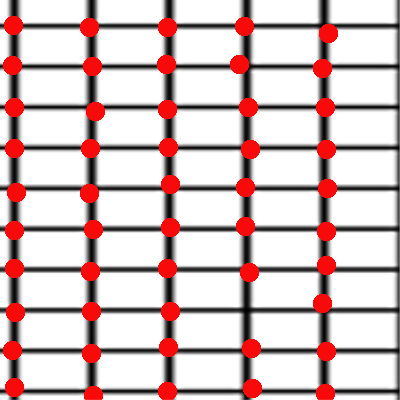# What is Marching Square? (with Implementation)

I haven't been blogging for a while but I had been planning to share a blog post on Marching Cube Algorithm for long. For those who don't know about Marching Cube algorithm, you can check out this Wikipedia-link.Circle using Marching Square
Marching Cube is simply a algorithm for surface reconstruction from a three dimensional scalar field. Pretty neat right? :D

Well, this post is not exactly about Marching Cubes, its about Marching Square, which will be a-lot helpful to grasp the overall concept of Marching Cube Algorithm.
Marching Cubes, as the name suggests, constructs 3D surface where as Marching Square works in 2D.

I have created a series of steps as algorithms to compute marching square:

1. Define a 2D scalar function.

This function can be a circle define by its equation:
x^2 + y^2 -  r^2 = 0

2. Define a grid size.Window with grids
Grid size is the size of grid with which our window is segmented.

3. Compute scalar values at each vertex of each grid-cell.Find Scalar Value at each vertex (denoted by red dots)

4. At each gridcell, check if isoline exists.
Scalar value > 0 means its outside the region ( circle )
Scalar value < 0 means its inside the region ( circle )
Scalar value = 0 means its at the region (circle)Green dots are the intersecting points. Red line is the isoline.
So, to check if isoline exists, we need to see if there's an edge with one scalar value positive and other negative. If there is any, then it means the region defined by scalar field intersects the grid cell and the line connecting those two intersecting point define a isoline.

5. If there's an intersection, use interpolation technique to find the exact intersection point.

After we find the edge that is intersect, we need to find the exact point of intersection. For this purpose we use interpolation. We can use the formula below:
P = P1 + (isovalue - V1)(P2-P1)/(V2-V1)
where P1 and P2 are the two points of intersected Edges. V1 and V2 are the scalar values at that point.

6. Save the intersection points ( two intersection point forms a isoline ) and draw them.

After collecting all the isoline, we can just draw them to get the region defined by scalar field. Here is the final image:With grid linesWithout grid lines

Now that you know how it works, you can easily implement it. The concept of Marching Square is pretty straight forward and this will be helpful to grasp the concept of Marching Cubes as well.

If you have any problems implementing it, you can refer the project here: Marching Square Implementation